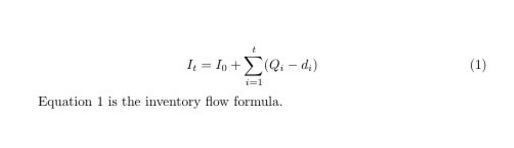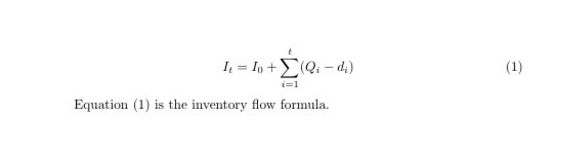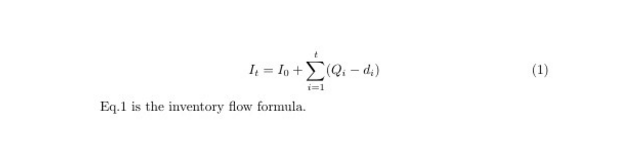# latex 引用公式

latex 运用引用功能，给公式添加标签 \label，就不用因为公式的编号变动而麻烦地更改大量数字了。

#### 1.  一般情况下，可以用 \ref 命令

\ begin{equation}
I_{t}=I_{0}+\sum_{i=1}^{t}(Q_{i}-d_{i})\label{con:inventoryflow}
\ end{equation}

Equation \ ref{con:inventoryflow} is the inventory flow formula.#### 2. 若要在引用的编号两边显示括号或中括号，则可以用 (\ref) 或 [\ref] 命令:

\ begin{equation}
I_{t}=I_{0}+\sum_{i=1}^{t}(Q_{i}-d_{i})\label{con:inventoryflow}
\ end{equation}

Equation (\ ref{con:inventoryflow}) is the inventory flow formula.

#### 3. 或加载 amsmath 工具包，使用 \eqref  命令：

\ begin{equation}
I_{t}=I_{0}+\sum_{i=1}^{t}(Q_{i}-d_{i})\label{con:inventoryflow}
\ end{equation}

Constraint \ eqref{con:inventoryflow} is the inventory flow formula.#### 4. 自定义引用样式，可以用 newcommand 自定义一个引用样式。

newcommand 函数的格式为：\newcommand{新命令}[参数数量][默认值]{定义内容}。默认值一般可以省略，只给出参数数量。#1表示参数内容。

\newcommand{\myref}{Eq.\ ref{#1}}
\ myref{con:inventoryflow} is the inventory flow formula.07-088330
10-122536

07-301万+
05-251万+
05-291万+
10-234083
12-031108
02-051万+
05-301万+
02-073万+
01-0511万+
01-16565
06-046608
09-076495
09-241603
11-091万+
07-081万+
01-1251
01-012240

### “相关推荐”对你有帮助么？

•非常没帮助
•没帮助
•一般
•有帮助
•非常有帮助¥2 ¥4 ¥6 ¥10 ¥20余额支付 (余额：-- )扫码支付获取中扫码支付点击重新获取扫码支付1.余额是钱包充值的虚拟货币，按照1:1的比例进行支付金额的抵扣。
2.余额无法直接购买下载，可以购买VIP、C币套餐、付费专栏及课程。余额充值